# skyplot

Plot satellite azimuth and elevation data

## Syntax

``skyplot(azdata,eldata)``
``skyplot(azdata,eldata,labeldata)``
``skyplot(status)``
``skyplot(___,Name,Value)``
``skyplot(parent,___)``
``h = skyplot(___)``

## Description

example

````skyplot(azdata,eldata)` creates a sky plot using the azimuth and elevation data specified as matrices in degrees. Azimuth angles are measured in degrees, clockwise-positive from the North direction. Elevation angles are measured from the horizon line with 90 degrees being directly up. For details about the sky plot figure elements, see Main Sky Plot Elements.```
````skyplot(azdata,eldata,labeldata)` specifies data labels as a string array with elements corresponding to each data point in the `azdata` and `eldata` inputs.```
````skyplot(status)` specifies the azimuth and elevation data in a structure with fields `SatelliteAzimuth` and `SatelliteElevation`.```

example

````skyplot(___,Name,Value)` specifies options using one or more name-value arguments in addition to the input arguments in previous syntaxes. The name-value arguments are properties of the `SkyPlotChart` object. For a list of properties, see SkyPlotChart Properties.```
````skyplot(parent,___)` creates the sky plot in the figure, panel, or tab specified by `parent`.```
````h = skyplot(___)` returns the sky plot as a `SkyPlotChart` object, `h`. Use `h` to modify the properties of the chart after creating it. For a list of properties, see SkyPlotChart Properties.```

## Examples

collapse all

Create a GNSS sensor model as a `gnssSensor` (Navigation Toolbox) System object™.

`gnss = gnssSensor; `

Specify the position and velocity of the sensor. Simulate the sensor readings and get status from visible satellites. Store the azimuth and elevation angles as vectors.

```pos = [0 0 0]; vel = [0 0 0]; [~, ~, status] = gnss(pos, vel); satAz = status.SatelliteAzimuth; satEl = status.SatelliteElevation; ```

Create random local elevation masks, with a maximum elevation of 30 degrees, to act as the local environment.

```rng(8) terrainMaskElevations = 30*rand(1,12); % elevations (degrees) terrainMaskEdges = [0 24 48 100 132 180 204 240 276 288 300 312 360]; % azimuth edges (degrees)```

Plot the satellite positions with the elevation masks.

`skyplot(satAz,satEl,MaskElevation=terrainMaskElevations,MaskAzimuthEdges=terrainMaskEdges); `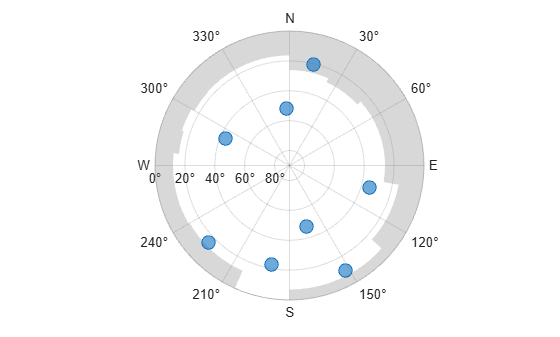Animate the trajectory of satellite positions over time from a GNSS sensor.

Initialize the sky plot figure. Specify the relevant time-stepping information.

`skyplotHandle = skyplot(0,0); `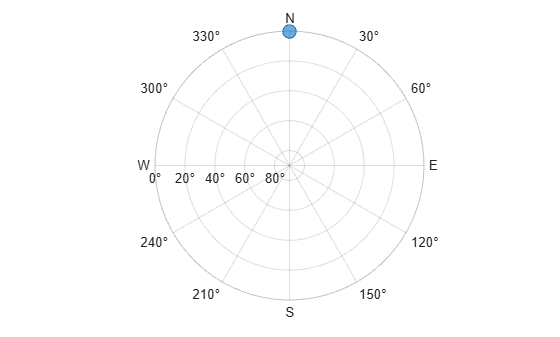```numHours = 12; dt = 100; numSeconds = numHours * 60 * 60; numSimSteps = numSeconds/dt;```

Create a GNSS sensor model as a `gnssSensor` (Navigation Toolbox) System Object™.

`gnss = gnssSensor('SampleRate', 1/dt); `

Iterate through the time steps and do the following:

• Simulate the sensor readings. Specify the zero postion and velocity for the stationary sensor.

• Store the azimuth and elevation angles as vectors.

• Set the `AzimuthData` and `ElevationData` properties of the `SkyPlotChart` handle directly.

```for i = 1:numSimSteps [~, ~, status] = gnss([0 0 0],[0 0 0]); satAz = status.SatelliteAzimuth; satEl = status.SatelliteElevation; set(skyplotHandle,'AzimuthData',satAz,'ElevationData',satEl); drawnow end ```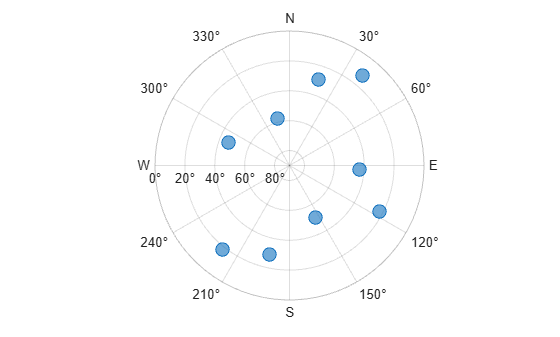Load the azimuth and elevation data from a logfile generated by an Adafruit® GPS satellite sensor. The data provided in this example contains the azimuth and elevation of each satellite and the pseudorandom noise (PRN) codes. Store these values as vectors.

```load('gpsHWInfo','hwInfo') satAz = hwInfo.SatelliteAzimuths; satEl = hwInfo.SatelliteElevations; prn = hwInfo.SatellitePRNs; ```

Separate the satellites based on the PRN codes. To correlate each position with a group, create a `categorical` array. For this set of satellites, only the ones with PRNs less than 32 are used in the positioning solution.

```isUnused = (prn > 32); group = categorical(isUnused,[false true],["Used in Positioning Solution" "Unused"]);```

Visualize the satellites and specify the categorical groups in the `GroupData` name-value argument. Specify the PRN as the label for each point. Show the legend.

```skyplot(satAz,satEl,prn,GroupData=group) legend('Used','Unused')```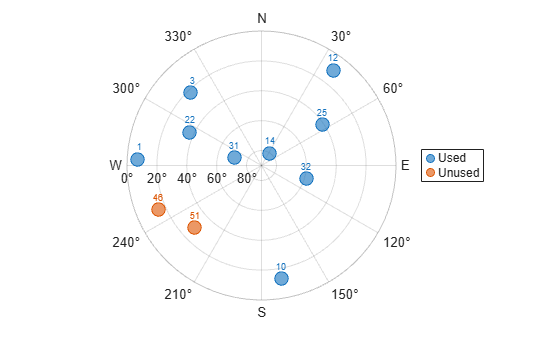Specify the receiver position, RINEX navigation file, mask angle, time step size, and number of hours of data to sample from the RINEX file.

```recPos = [42 -71 50]; navfile = "GODS00USA_R_20211750000_01D_GN.rnx"; maskAngle = 25; dt = 60; % seconds numHours = 4;```

Read the navigation file, and get the GPS data of all satellites captured in the file.

```data = rinexread(navfile); [~,satIdx] = unique(data.GPS.SatelliteID); navmsg = data.GPS(satIdx,:);```

Set the starting time to the initial time of the navigation message. Then, create the time vector `t.`

```startTime = navmsg.Time(1); secondsPerHour = 3600; timeElapsed = 0:dt:(secondsPerHour*numHours); t = startTime + seconds(timeElapsed);```

Initialize vectors for azimuth and elevation. Then, collect azimuth and elevation data at times `t` for all satellites.

```numSats = numel(navmsg.SatelliteID); allAz = NaN(numel(t),numSats); allEl = allAz; for idx = 1:numel(t) [satPos,~,satID] = gnssconstellation(t(idx),RINEXData=navmsg); [az,el,vis] = lookangles(recPos,satPos,maskAngle); allAz(idx,:) = az; allEl(idx,:) = el; end```

Mark all satellites below the horizon with an elevation less than `0` as missing.

`allEl(allEl < 0) = missing;`

Display the satellite trajectories as an animation by creating a skyplot and updating the `AzimuthData` and `ElevationData` properties.

```figure sp = skyplot(allAz(1,:),allEl(1,:),satID,MaskElevation=maskAngle); for idx = 1:size(allAz, 1) set(sp,AzimuthData=allAz(1:idx,:),ElevationData=allEl(1:idx,:)); drawnow limitrate end```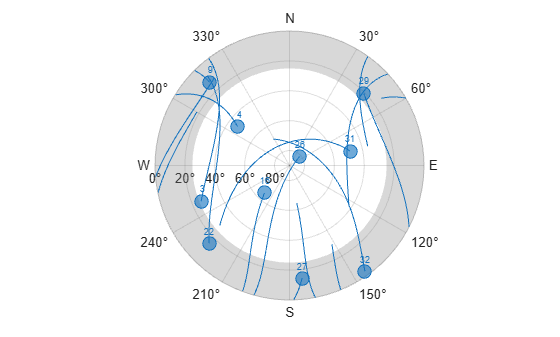## Input Arguments

collapse all

Azimuth angles for visible satellite positions, specified as an n-element vector of angles or t-by-n matrix of angles. n is the number of visible satellite positions in the plot, and t is the number of time steps of the satellites. Azimuth angles are measured in degrees, clockwise-positive from the north direction.

Example: `[25 45 182 356]` specifies the azimuth angles for four satellites at one time step.

Data Types: `double`

Elevation angles for visible satellite positions, specified as an n-element vector of angles or t-by-n matrix of angles. n is the number of visible satellite positions in the plot, and t is the number of time steps of the satellites. Elevation angles are measured from the horizon line with 90 degrees being directly up.

Example: `[45 90 27 74]` specifies the elevation angles for four satellites at one time step.

Data Types: `double`

Labels for visible satellite positions, specified as an n-element string array. n is the number of visible satellite positions in the plot.

Example: `["G1" "G11" "G7" "G3"]`

Data Types: `string`

Satellite status, specified as a structure array with fields `SatelliteAzimuth` and `SatelliteElevation`. Typically, this status structure comes from a `gnssSensor` (Navigation Toolbox) object, which simulates satellite positions and velocities.

Example: ```gnss = gnssSensor; [~,~,status] = gnss(position,velocity)```

Data Types: `struct`

Parent container, specified as a `Figure`, `Panel`, `Tab`, `TiledChartLayout`, or `GridLayout` object.

## Output Arguments

collapse all

Sky plot chart, returned as a `SkyPlotChart` object, which is a standalone visualization. Use `h` to set properties on the sky plot chart. For more information, see SkyPlotChart Properties (Navigation Toolbox).

collapse all

### Main Sky Plot Elements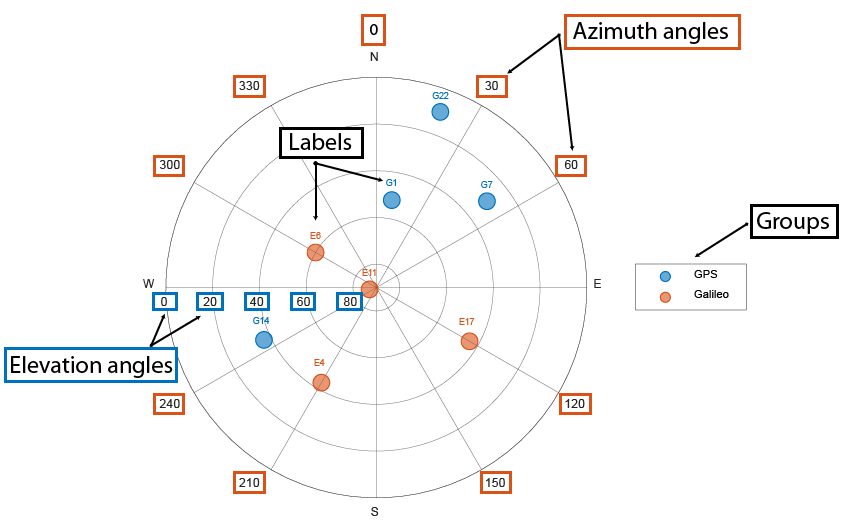The main elements of the figure are:

• Azimuth axes — Specified by the `azdata` input argument, azimuth angle positions are measured clockwise-positive from the North direction.

• Elevation axes —Specified by the `eldata` input argument, elevation angle positions are measured from the horizon line with 90 degrees being directly up.

• Labels — Specified by the `labeldata` input argument as a string array with an element for each point in the `azdata` and `eldata` vectors.

• Groups — Specified by the `GroupData` (Navigation Toolbox) property, a `categorical` array defines the group for each satellite position.

### Standalone Visualization

A standalone visualization is a chart designed for a special purpose that works independently from other charts. Unlike other charts such as `plot` and `surf`, a standalone visualization has a preconfigured axes object built into it, and some customizations are not available. A standalone visualization also has these characteristics:

• It cannot be combined with other graphics elements, such as lines, patches, or surfaces. Thus, the `hold` command is not supported.

• The `gca` function can return the chart object as the current axes.

• You can pass the chart object to many MATLAB® functions that accept an axes object as an input argument. For example, you can pass the chart object to the `title` function.

## Version History

Introduced in R2021a

expand all